# F D C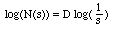## Fractal Dimension Calculator

Written by Paul Bourke
February 2003

Introduction

FDC estimates the fractal dimension of an object represented as a black and white image where the object to be analysed is assumed to be made up of the black pixels. This is accomplished by an algorithm called "box-counting". The application provided here is a upgrade to a very popular application written for the Macintosh back in the early 90's. This upgrade is provided for Mac OS-X (as a UNIX application). An earlier application (no longer available) along with the documentation can be found here, in what follows it will be assumed the documentation for the earlier version has been read.

Brief theory

Consider a line, if we subdivide the line in half then it takes two bits to recreate the original line. If we subdivide the line into 4 pieces it takes 4 of them to cover the line. We can write this generally, if we have a line segment of length "s' then the number of segments that will cover the original line is given by N(s) = (1/s)1.

Consider a square, if we subdivide it into smaller squares each with 1/2 the side length then it takes 4 of these smaller pieces to form the original square. If we subdivide the square into smaller squares each with 1/4 of the side length then it takes 16 of them to form the original square. As above we can write an expression for the number of pieces we need of size "s" to cover the original square, it is N(s) = (1/s)2.

Repeat for a cube....N(s) = (1/s)3.

The exponents 1, 2, and 3 in the above examples are fundamental to our concept of the dimension involved. This can be generalised to N(s) = (1/s)D where D is the dimension, an integer as above but it need not be. If we take logarithms of both sides we have log(N(s)) = D log(1/s), in order words we can estimate the dimension by plotting log(N(s)) against log(1/s) the slope of which is the dimension, if it isn't an integer then it's a fractional (fractal) dimension.

Screen dumps

A side goal for this project was to see to what extent graphical software could be written and which would compile on multiple UNIX based system including Mac OS-X. The aim was to create a single source version rather than have any special case code for any particular platform. To this end, all the graphics are created using OpenGL and the GLUT interface, all dialogs and user IO is implemented with the Forms (xforms) library. So, it is assumed that OpenGL and X are available, a fairly common situation.

 Mac OS-XLinux (SUSE, KDE)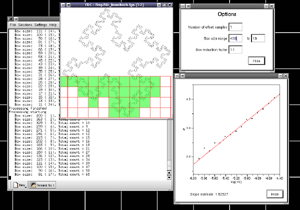Dec Alpha (OSF, Tru64)SGI (Irix)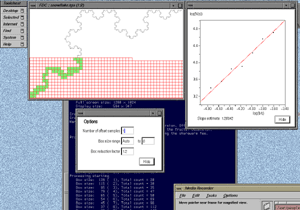FDC is a Mac OSX application that can be freely downloaded for evaluation. It is fully functional except that offset sampling (a critical feature for good estimates of the fractal dimension) isn't enabled. This allows prospective users to check the application runs on their system and performs as expected before investing in a version which will compute more reliable estimates of the fractal dimension. If you have any problems with these demo version please contact the author. To obtain the full version with offset sampling enabled the shareware fee of US\$30 is payable, preferably through PayPal.

Note than from Lion onward you may need to install X-Windows separately, it is recommended you use XQuartz. Also, you will need a 3 button mouse since the right mouse button is used for the menus. This can be achieved by enabling the secondary mouse button on the Apple mice.

 Demo versions:    OSX Leopard: fdc_leopard.zip    OSX Lion: fdc_lion.zip    OSX MountainLion: fdc_mountainlion.zip    OSX Yosemite: fdc_yosemite.zip    OSX Sierra: fdc_sierra.zip BuySample TGA files: tgasamples.tar.gz(Note: fdc only reads 24 or 32 bit TGA files)

Running FDC

FDC can be run in either graphical mode where the user chooses options and gets graphical feedback on the process or it can be run quietly. FDC will present a window with the current image (it will be empty, white), from here you can use the right mouse button (control click on a Mac with only a single mouse button) to show the menus from where you can open a TGA file (24 or 32 bit), set options, start and stop the processing, display the graph, and save the results for further analysis.

The full list of command line options for FDC can be viewed by typing "fdc -h", at the time of writing they are as follows.

```Usage: fdc [options]
General options
-h      this text (help)
-i s    load input TGA file
-bw     invert image
-q      quiet, command line mode
-p      start processing immediately
Box options
-n  n   number of offset samples, default: 10
-b1 n   start box size (default: -1)
-b2 n   end box size (default: 6)
-bf n   box reduction factor (default: 1.2)
Region options
-sl     left boundary (default: 0)
-sr     right boundary (default: image width)
-sb     bottom boundary (default: 0)
-st     top boundary (default: image height)
```

So for example the command line to run the analysis on the image "snowflake.tga" (supplied in the FDC distribution) quietly with the results written to standard output, using box sizes that range from 200 to 8 in factor steps of 1.2 with 100 offset samples might be as follows.

```   fdc -q -i snowflake.tga -b1 200 -b2 8 -n 100 -bf 1.2 > snowflake.dat
```

The results in "snowflake.dat" will be something like the text on the left below. Where the first column is the box size (s), the second column is the minimum number of covering boxes (N), the third column is log(1/s), and the last column is log(N). The estimate of the fractal dimension is the slope of this graph. If the application is run in graphical mode, at the end of the processing the graph window will look something like the image on the right.

 ```200 11 -5.29832 2.3979 166 15 -5.11199 2.70805 138 17 -4.92725 2.83321 114 22 -4.7362 3.09104 95 28 -4.55388 3.3322 79 37 -4.36945 3.61092 65 43 -4.17439 3.7612 54 61 -3.98898 4.11087 45 74 -3.80666 4.30407 37 92 -3.61092 4.52179 30 112 -3.4012 4.7185 24 179 -3.17805 5.18739 20 208 -2.99573 5.33754 16 284 -2.77259 5.64897 13 385 -2.56495 5.95324 10 492 -2.30259 6.19848 8 698 -2.07944 6.54822 ``` Note that the theoretical fractal dimension for the snowflake curve is log(4)/log(3) = 1.262.The processing will generally run faster if the options and graph window are hidden. It will run significantly faster if run in "quiet" mode using the "-q" command line option. This is particularly important if a large number of offsets are being used.

Box size range

The default range of box sizes is usually optimal but not always so. They should only be changed when you have some experience and understanding of the processes involved. The following graph is the result of performing the box counting where the box size ranged from being 5 times the width (or height) of the image to 1 pixel. For successful choice of the box size range it is important to understand the regions of the graph illustrated here.Testing

The following are some shapes for which the exact fractal dimension is known, these can test the convergence and the dimension estimated using this software. Note that for the examples below which have a fractal dimension one doesn't expect a perfect match to theoretical because the image is only an approximation to the real fractal form. Note that since FDC assumes the object doesn't exist outside the image area, the estimates for the Sierpinski Carpet are particularly sensitive to this.

 Snowflake Dimension: log(4)/log(3) = 1.262 Calculated: 1.235 (1 offset) Calculated: 1.277 (10 offsets) Calculated: 1.280 (100 offsets)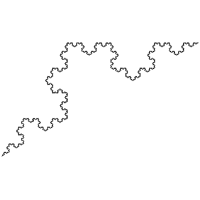Koch island Dimension: 1.5 Calculated: 1.441 (1 offset) Calculated: 1.450 (10 offsets) Calculated: 1.480 (100 offsets)Sierpinski Carpet Dimension: log(8)/log(3) = 1.893 Calculated: 1.818 (1 offset) Calculated: 1.832 (10 offsets) Calculated: 1.840 (100 offsets)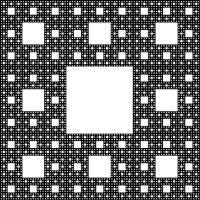Line Dimension: 1 Calculated: 0.975 (1 offset) Calculated: 0.988 (10 offsets) Calculated: 0.993 (100 offsets)Solid Dimension: 2 Calculated: 1.929 (1 offset) Calculated: 1.950 (10 offsets) Calculated: 1.964 (100 offsets)Sierpinski Gasket Dimension: log(3)/log(2) = 1.585 Calculated: 1.672 (1 offset) Calculated: 1.594 (10 offsets) Calculated: 1.590 (100 offsets)It is possible to analyse just a portion of the image. For example

`   fdc -sr 700 -sl 400 -sb 300 -st 600 -i gasket.tga`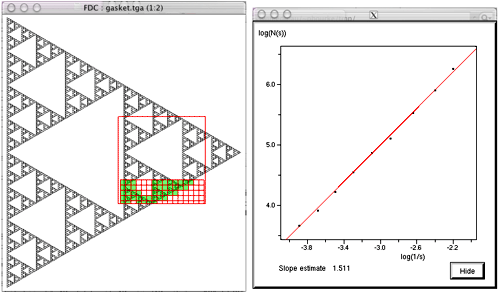It is anticipated that in normal operation the area of interest will be chosen in an image editing page prior to analysing the fractal dimension. The ability to process just a region of the image is intended to be used with automatic scripts to estimate the fractal dimension locally across the image, for example, when the dimension is expected to vary across the image. The approach that might be used is to slide a rectangular region across the image estimating the dimension at each position, these estimates can then be combined to form an image showing the dimensional variation. This is similar to a sliding window used in signal processing to calculate the Fourier spectrum variation of a time series. There are two variables involved, the width of the window and the distance between two successive window positions. Typically there is significant overlap between the windows. The image below is formed by analysing a gasket (image size 840 by 950) with a window width of 200 and a window step size of 20.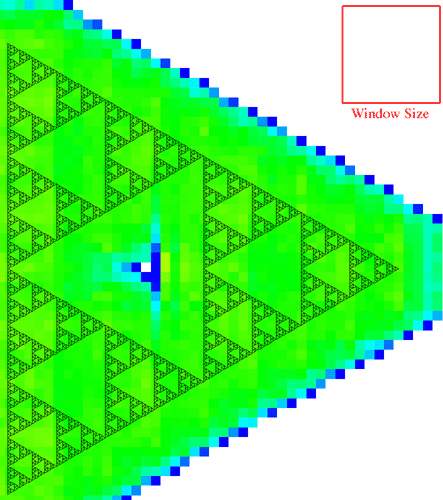The colour scale is a blue to green to red colour ramp where blue is 1 and red is 2. The correct dimension in this case is 1.585 hence the green appearance. The white region is where the window didn't intersect with any part of the image.The window size and stepping are chosen based upon a trade-off between temporal resolution and reliability of the dimension estimate. A large window gives good estimates but low resolution (ability to detect local variations in the dimension), while a small window gives good resolution but poorer estimates. In the example above there are good estimates but low resolution. In the example below a window of 50 is used with the same stepping size of 20, here there is better resolution but poorer estimates.Please note that the above colour plots of the sliding window dimension estimates is not part of the FDC package. FDC creates the data for the above but it is up to user to turn that data into a suitable form for visual inspection.

Applications of this software

Using Box Counting Techniques for Measuring Shape of Colonies of Filamentous Micro-organisms. Jacques Soddell and Robert Seviour, Complexity International (1995) 2

Viscosity of Venusian Lava Flows: Constraints from Fractal Dimension and Chemical Composition. W. A. Pike, H.M. Frey, A.E. Krull, E.B. Grosfils, M.S. Gilmore, L.A. Reinen, and S.J. Kozak. Lunar and Planetary Sciences XX1X

GIS based Compactness measurement Using Fractal Analysis. Jeffrey L. Knight. George Mason University Fairfax, Virginia

The Box-Counting: Critical Study. M. Nezádal, O. Zmeskal, M. Buchnicek. Technical University of Brno, Faculty of Chemistry, Institute of Physical and Applied Chemistry, Purkynova 118, 612 00 Brno, Czech Republic

Fractal Properties of the Thyrotropic Feedback Control Implications of a Non-linear Model Compared with Empirical Data. J. W. Dietrich, A. Tesche, C. R. Pickardt and U. Mitzdorf. Cybernetics and Systems, 2002

Measuring Ocular Redness: First order (luminance & chromaticity) measurements provide more information than second order (spatial structure) measurements. T. Simpson, A. Chan, D. Fonn. Center for Contact Lens Research, School of Optometry, University of Waterloo.

Incident Detection by Fractal Dimension Analysis of Loop Detection Data. Kim Thomas and Husseun Dia. 22nd Conference of Australian Institutes of transport Research (CAITR-2000), Ursula College, ANU Campus, Canberra, Australia.

How big is the Milky Way? +plus issue 15. Toby O'Neil

Advances in the implementation of the box-counting method of fractal dimension estimation. K Foroutan-pour, P Dutilleul, DL Smith. Applied Mathematics and Computation
Vol. 105 No. 2-3 NOV 1999

References

Texture Description and Segmentation through Fractal Geometry. J.M. Keller, S.Chen, R.M. Crownover. Computer Vision, Graphics, and Image Processing. 45, 150-166, (1989).

The Fractal Geometry of Nature. B.B. Mandelbrot. San Francisco, Freeman.

Fractals and Disordered Systems. A. Bunde, S. Havlin. Springer Verlag, Berlin, Heidelberg.

The Infinite Number of Generalised Dimensions of Fractals and Strange Attractors. H.G.E.Hentschel, I. Procacaccia. Physica (Amsterdam) 8D, 435 (1983)

Determination of Fractal Dimension for Geometrical Multifractals. T. Tel, A. Fulop, T. Vicsek. Physica A, 159, 155-166 (1989)

# Ruler or Compass Dimension

Written by Paul Bourke
October 1998

Update June 2017, see below for an implementation for vector data.

While this application is not generally available, if it is of interest then please contact the author by email. The author is also available for consulting and bespoke software development in this area.

Introduction

A "standard" method of estimating the length of a curve on a plane is to count how many ruler lengths it takes to move from the start of the curve to the end. Since the ruler has a finite length, the details of the curve that are smaller than the ruler get skipped over and therefore the length we measure is normally less than the real length. This is illustrated below for two rulers (red) of different length being used to estimate the length of the curve in black.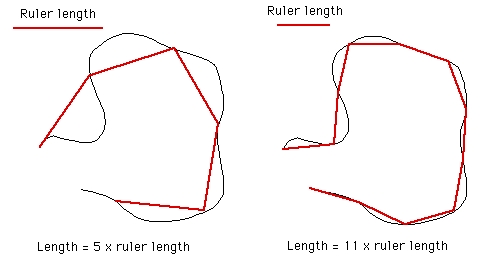Intuitively we believe that as our ruler gets shorter, our estimate of the real length improves and increases. The estimate of the length on the right image above is closer to the true length because it follows the curve more closely.

This improved estimate as the ruler length decreases is certainly true for many curves (1 dimensional objects) but it is not true for others. Such curves are referred to as fractals and they have what is known as a fractal dimension between 1 and 2. Dimension measured in this way is referred to as the Hausdorff Besicovitch dimension.

Perhaps the most often quoted example is "How long is the coast of Britain?". One could measure the length using a map and a compass set to say a 50km radius. A better estimate would be achieved by using smaller and smaller compass radii, each one revealing more and more detail. One could go further and have someone walk around the coast, they would still make decisions on how closely they followed the beach. As one became more and more "precise" one would find more and more detail about which to become precise about. As with most fractals in the real world this process and therefore the fractal nature of the object is only appropriate over some limited range of scales.

There are many such examples in nature where the relationship between the measured length and the ruler length is not linear, ie: 1 dimensional. The outline of mountain ranges, clouds, and Brownian motion are another examples.

The ruler or compass dimension of a curve is computed from the relationship between the length of a ruler and the number of rulers required to measure the length of the curve. This is given by

N(l) = c l-D

where c is some constant. Taking logs of both sides

log(N(l)) = log(c) - D log(l)

So if we plot log(N(l)) against log(l) for a range of values of l, the slope will be an estimate of the fractal dimension D.

Implementation

The software described here estimates the length of a curve by measuring it with successively shorter ruler lengths. The results when plotted as described above give an estimate of the fractal dimension of the curve.

 The software requires a representation of the curve, this is as a file of (x,y) coordinates. The file format is straightforward, it is a series of x,y pairs as either integers of floating point numbers, each number and each pair are separated by any "white space" character (tab, space, carriage return. linefeed, etc). The first few lines of such a file format are shown on the right. ``` 17.3205 100 25.9808 105 34.641 100 34.641 90 43.3013 95 51.9615 90 51.9615 100 : : : : ```

Note: only continuous curves are possible in this implementation.

The range of line lengths is varied in powers of sqrt(2) from half the diagonal length of the bounding box of the curve to either
- 1/512'th of the diagonal length, or
- the length of the shortest line segment
which ever occurs first.

As the software reduces the ruler it graphically shows the results. The diagrams below are screen snapshots for the first 6 rulers analysing Brownian (1/f2) noise. The black line is the curve being analysed, the blue lines are the ruler lines, and the red lines are the compass radii.

 Ruler 1 (longest)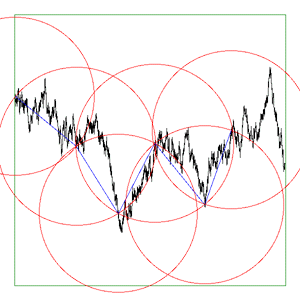Ruler 2Ruler 3Ruler 4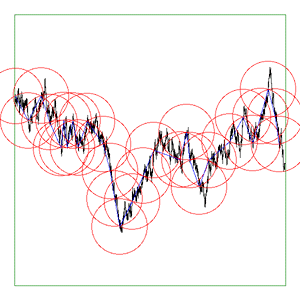Ruler 5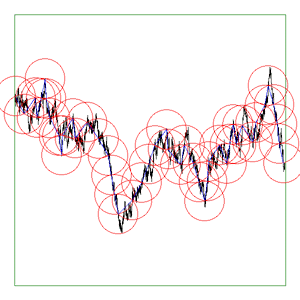Ruler 6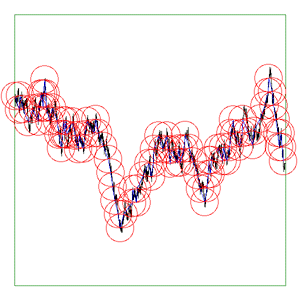The graph of log(l) vs log(N(l)) for the above analysis is given below. The expected dimension is 1.5 (Hurst exponent H = 0.5, D = 2 - H) Before the software is used to compute the fractal dimension of curves we don't know the dimension of, it should be tested with curves for which we do know the dimension. A few examples are given below.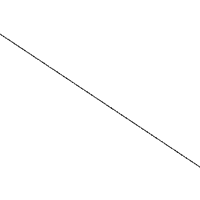Expected: D = 1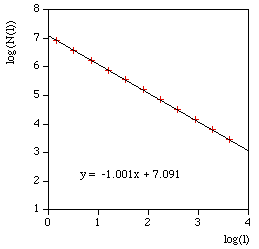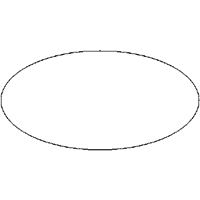Expected: D = 1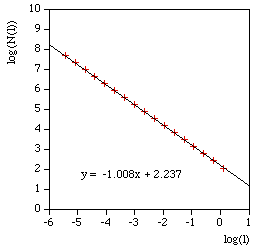Expected: D = log(4)/log(3) = 1.26....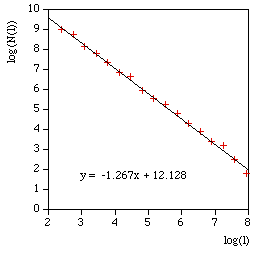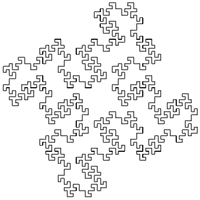Expected: D = log(8)/log(4) = 1.5The above are applied to image representation of curves, this can be limiting for many datasets that might have both large scale and fine grain detail thus requiring very large image resolutions to effectively be represented as an image. There are other problems in the reliability of the compass dimension estimates arising from rasterisation of vector data. The software described here (vcompassdim) computes the compass dimension for vector data in 2D.

```Usage: vcompassdim [options] filename
Options
-w n    Size of output image files, default: do not create
```

The data is conveyed to the software through a text file consisting of x,y pairs, each number and pairs can be separated by any white space character but usually it is a space between x and y and a carriage return before the next pair. The first few lines of an example data file might be as follows

```17.3205 90
17.3205 100
25.9808 105
34.641 100
34.641 90
43.3013 95
51.9615 90
51.9615 100
60.6218 105
51.9615 110
51.9615 120
60.6218 125
:
:
:
```

As per the early fractal dimension estimators, before applying to real data the code should be carefully tested against curves with known dimension. One such curve is the Koch curve with a fracal dimension of log(4)/log(3) = 1.26. A vector form of that curve is generated and the graph of log(l) vs log(N(l)) and linear curve fit shown below.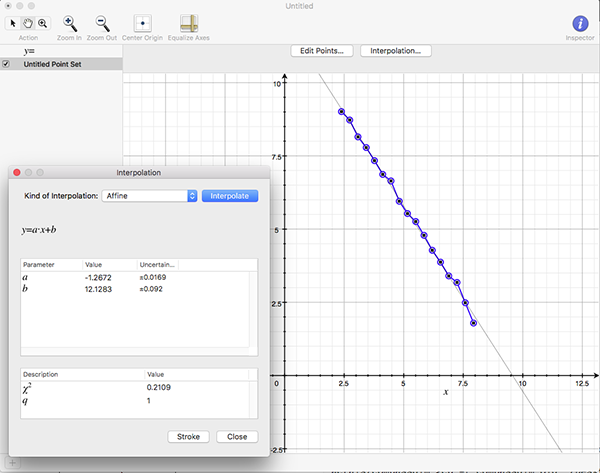As a visual check the software optionally outputs an image for every compass length. As example is shown below, the black line is the curve read from the data file, the blue lines are the rulers following the curve, and the red circles are the compass arcs.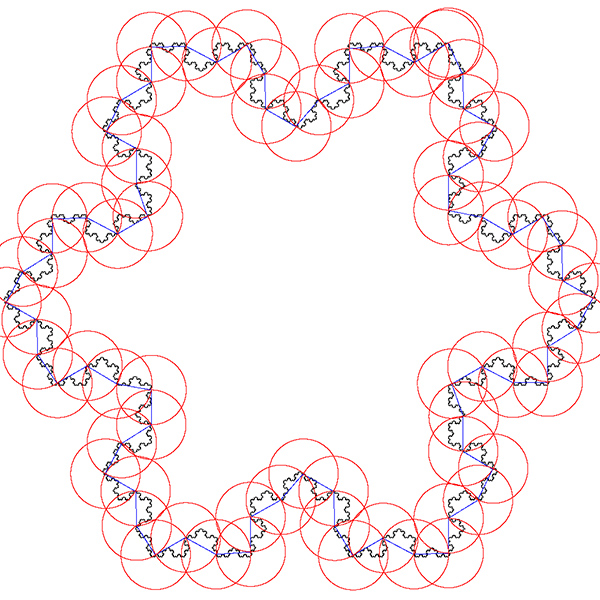Another example might be to apply to a space filling curve, where the compass dimension should approach 2. An example is below.The software writes the log(l) and log(N(l)) as text in 4 columns as follows

```l	N(l)	log(l)	log(N(l))
857.369	7	6.75387	1.94591
606.251	21	6.40729	3.04452
428.684	26	6.06072	3.2581
303.126	55	5.71415	4.00733
214.342	116	5.36757	4.75359
151.563	242	5.021	5.48894
107.171	363	4.67443	5.8944
75.7814	810	4.32785	6.69703
53.5855	2186	3.98128	7.68983
37.8907	3280	3.63471	8.0956
26.7928	6561	3.28813	8.7889
18.9454	19683	2.94156	9.88751
13.3964	24907	2.59499	10.1229
9.47268	59049	2.24841	10.9861
6.69819	68890	1.90184	11.1403
4.73634	118098	1.55526	11.6793
```

And finally the compass dimension is the slope as shown below.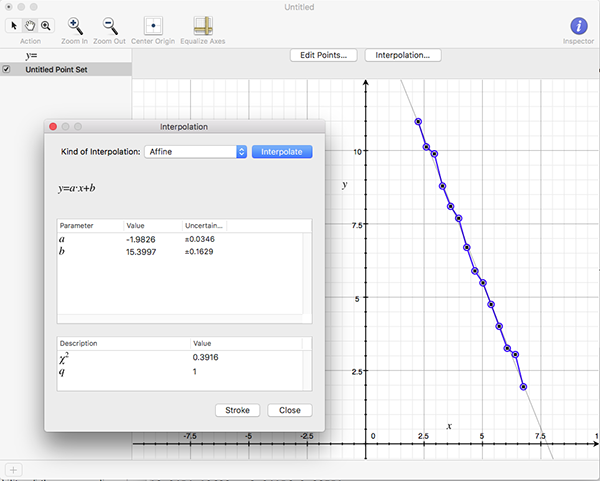References

Andrew Harrison. Fractals In Chemistry, Oxford Chemistry Primers. Oxford Science Publications, 1995

T.G. Smith Jr., W.B. Marks, G.D. Lang, W.H. Sheriff Jr., and E.A. Neal. A Fractal Analysis of Cell Images. Journal of Neuroscience Methods, Vol 27, pp 173-180, 1989

Heinz-Otto Peitgen. Fractals in the Classroom, Part 1. Springer-Verlag, 1991, pp 240

Heinz-Otto Peitgen, Dietmer Saupe. The Science of Fractal Images. Springer-Verlag, pp 61, 1985

# Lacunarity

Written by Paul Bourke
April 2004

Lacunarity was initially introduced as a means of further classifying fractals and textures which had the same fractal dimension and a very different visual appearance. Lacunarity is a measure of how the fractal fills space, if the fractal is dense the lacunarity is small, the lacunarity increases with coarseness.

Method 1: (Voss)

Let P(m,r) be the probability that a box of size r contains m black pixels. Define M1(r) and M2(r) as follows

 r2 M1(r) =m P(m,r) m=1

and

 r2 M2(r) =m2 P(m,r) m=1

then

 M2(r) - M12(r) L(r) =M12(r)
Method 2:

Calculate the mean M(r) and variance V(r) of the number of black pixels in each box size r.

 V(r) L(r) =M2(r)
Notes

• These two definitions give identical results.

• The object being studied is assumed to be made up of black pixels, all non object pixels are white.

• The value for a 1 pixel box (r = 1) reduces to the ratio of the white to black pixels, most easily derived from the equations for method 1.

• Only include those boxes which are totally enclosed within the boundary of the image.

• As the number of occupied pixels (black) tends to 0 the lacunarity tends to infinity, so sparse data will generally have higher lacunarity curves.

• If lacunarity is plotted as log(L(r)) vs log(r) then fractals will have straight line plots, in other words, they have similar appearance across scales.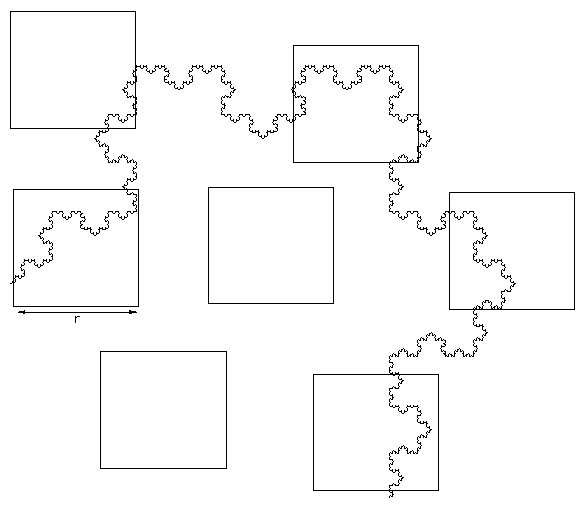Examples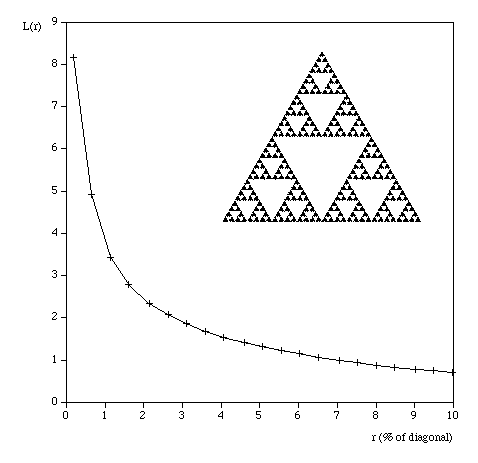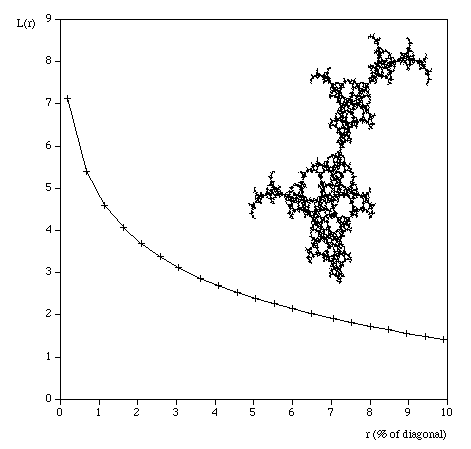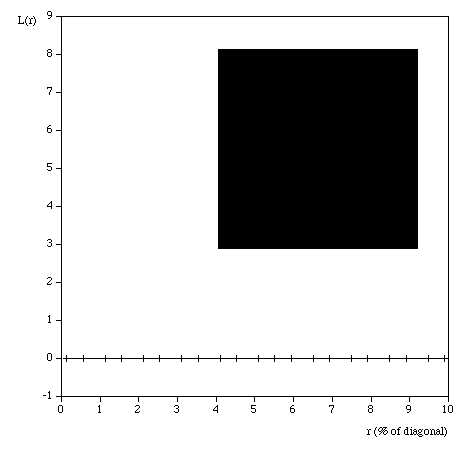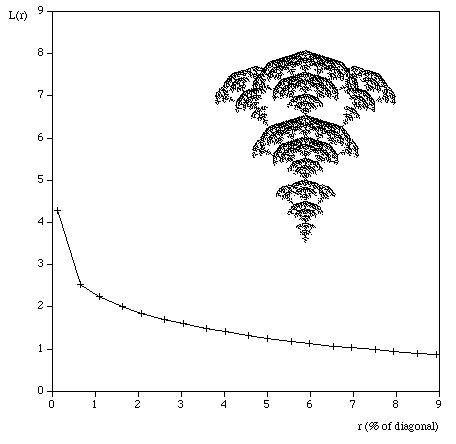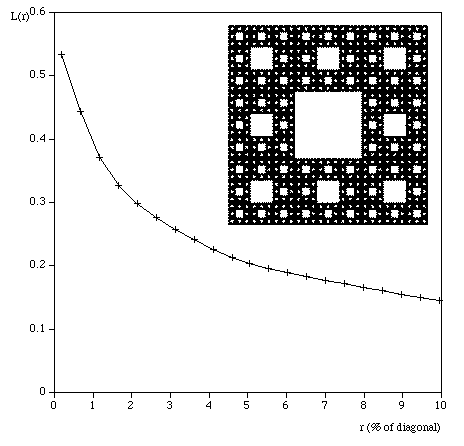References

Texture Description and Segmentation through Fractal Geometry. J.M. Keller, S. Chen, R.M. Crowover. Computer Vision, Graphics, and Image Processing, 45, 150-166 (1989)

Characterisation and measurement to scaling phenomena in disordered systems. R. Voss, R. Pynn and A Skjeltorp, (Ed), New York, 1986.

Fractal Methods and Results in Cellular Morphology. G.D. Lange and W.B. Marks. J. Neurosci, 69, 1123-1126 (1996)

Lacunarity calculation in the true fractal limit. D.A.Fabio, A. Reis, and R. Riera. J. Phys. A. Math, 27, 1827-1835 (1994)

Three-dimensional image analysis and fractal characterization of kidney arterial vessels. M. Sernetz, J. Wubbeke, and P. Wiezek. Physica A, 191: 13-16. (1992)

# Multifractal spectrum

Written by Paul Bourke
January 2004

Box Mass

Let N(r) be the total number of boxes (non overlapping) of size length r covering the object. Let Mi(r) be the mass within the i'th box. Define Z(q,r) as follows.

 N(r) Z(q,r) =[ Mi(r) ]q i=1

The multifractal dimension D(q) is given by

(q - 1) D(q) = slope of log(Z(q,r)) vs log(r)

q ranges from -infinity (concentrates on less dense regions) and +infinity (concentrates on dense regions).

For q = 0 this reduces to standard box dimension.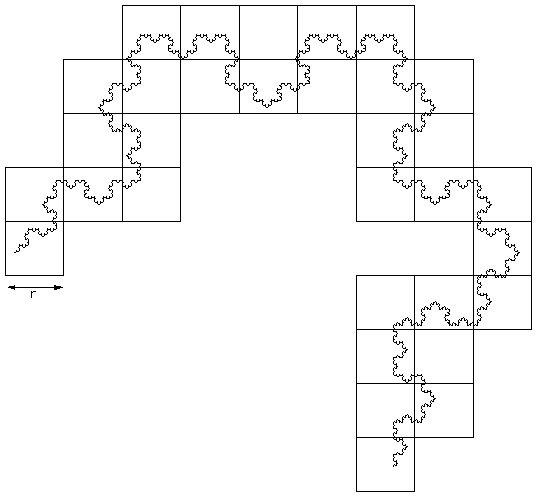Consider all circles of radius r that have their center on the object. Let Mi(r) be the mass within the i'th circle, and the total number of circles of radius r is N(r). Then Z(q,r) is defined as

N(r)
Z(q,r) =[ Mi(r) ]qN(r)
i=1

The multifractal dimension D(q) is given by

q Q(q) = slope of log(Z(q,r)) vs log(r)Examples

 Gasket Fractal dimension: log(3) / log(2) = 1.585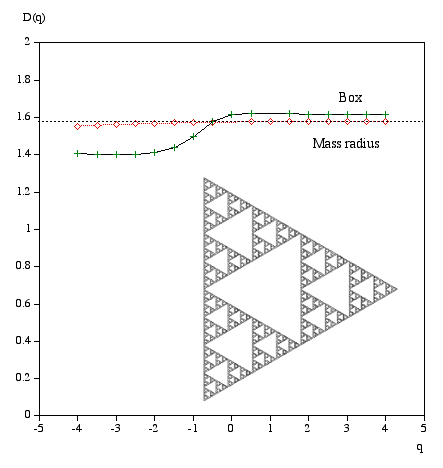Snowflake Fractal dimension: log(4) / log(3) = 1.262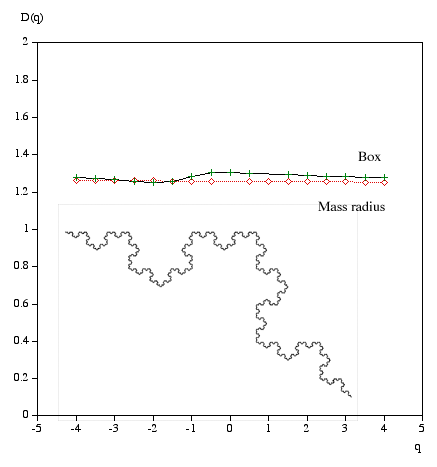WeedLine Dimension = 1Solid black Dimension: 2MM D-infinity = log(17)/log(5) = 1.760 Dinfinity = log(17/4)/log(5/2) = 1.570NN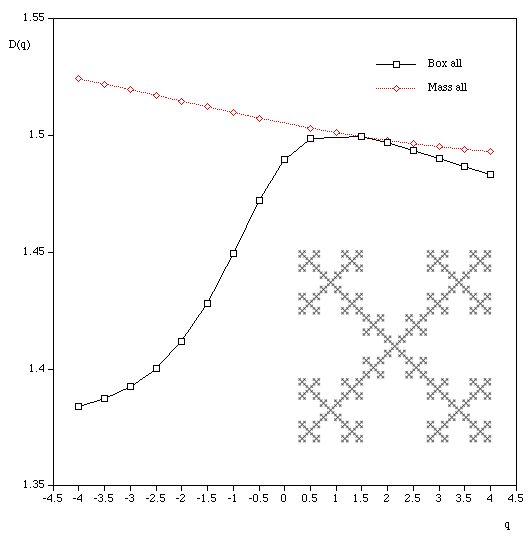DLARandom 1000x1000 pixels 100000 random points. Uniformly distributed on both axes.Random 1000x1000 pixels 500000 random points. Uniformly distributed on both axes.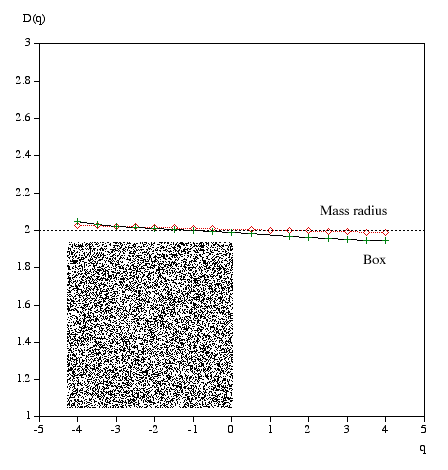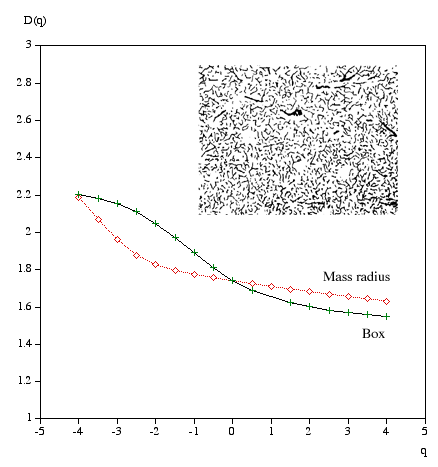References

Multifractal Characterization of Soil Particle-Size Distributions. A.N.D. Posadas, D. Gimenez, M. Bittelli, C.M.P. Vaz, and M. Flury. Soil Sci. Soc. Am. J. 65:1361–1367 (2001)

Direct Determination of the f(alpha) Singularity Spectrum. A. Chhabra and R.V. Jensen. Physical Review Letters, 62, March 1989, #12

Multifractal features of random walks on random fractals. A. Bunde, S. Havlin, H.E. Roman. Physical Reviewm A 42, 6274 (1990)

Multifractal phenomena in physics and chemistry. H.E. Stanley and P. Meakin. Nature, 335, 405 (1988)

Measuring the strangeness of strange attractors. P. Grassberger and I. Procaccia. Physica, Amsterdam, 9D, 189 (1983)

Scaling in financial prices: IV Multifractal Concentration. M.B. Mandelbrot. Quantitative Finance, Vol 1, 641-649 (2001)

Are Neurons multifractals. E. Fernandez, J.A. Bolen, et al. Journal of Neuroscience Methods, 89, 151-157 (1999)

Physical mechanisms underlying neurite outgrowth: a quantitative analysis of neuronal shape. Caserta, F., et al. Physical Review Letters, 1990. 64(1): p. 95-9

# Recurrence plots

Written by Paul Bourke
January 1998

Recurrence plots are used to reveal non-stationarity of a series as well as to indicate the degree of aperiodicity. They were first proposed by Eckmann et al around 1986 in order to study phase space orbits. For example, for a stationary system the recurrence plot should generally be homogeneous along the diagonal.

Consider a series x with N terms

x0, x1, x2, x3, x4, x5, .... xi, ..... xN-1

Form all the vectors yi of dimension (length) D with lag (delay) d.

yi = ( xi, xi+d, xi+2d, .... )

D >= 2 and d >= 1

This is normally referred to as embedding x in dimension D with lag d.

The recurrence plot is formed by comparing all embedded vectors with each other, and drawing points when the distance between two vectors is below some threshold. More precisely, we draw a point at coordinate (i,j) if the i'th and j'th embedded vectors are less than some distance r apart, eg: a point is drawn if

||yi - yj|| < r

i is plotted along the horizontal axis, j on the vertical axis.

 If the recurrence plot contains a homogeneous but irregular distribution of points then the series is mostly stochastic. For example, for a random series (white noise with mean = 0, standard deviation = 1) the recurrence plot is as shown below (d = 2, h = 1, r = 0.05) Note that the recurrence plot is diagonally symmetric since the distance of the i'th embedded vector to the j'th embedded vector is the same as the distance of the j'th to the i'th. Also, there is a diagonal line where i = j (the distance between the vectors is 0 and hence always less than the threshold)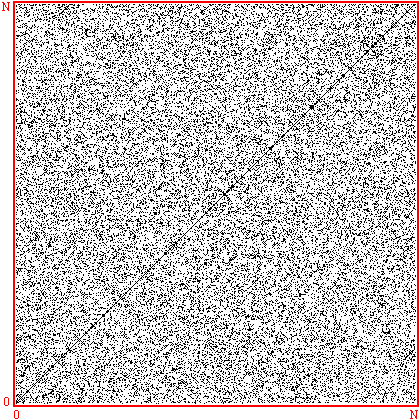Long straight parallel lines indicate a periodic series. For example the following is the recurrence plot for a sinusoid, the distance between the diagonal lines is the period. Horizontal and vertical white lines or bands are associated with states that don't occur often. Horizontal and vertical black lines indicate states that remain unchanged for periods of time.One of the difficulties is determining an appropriate distance r. In the software developed here and listed below, the user can select a value of r, the software then creates 3 recurrence plots with r/2, r, and 2r. Another approach is to show all radii and to illustrate this with a colour recurrence plot where the range of radii are mapped onto different colours. In the example on the right the same sinusoid is used as in the last example and the radius r is mapped onto a blue to red colour map (blue = small r, red = large r).For a chaotic series on the right the diagram is more complicated. There are brief periodic pieces (short diagonal strips). The time series used below is the logistic equation operating in the chaotic region, namely x = 4 * xn-1 ( 1 - xn-1 )Significant density changes near the diagonal is an indication of non-stationarity. The following plot is for a linearly swept chirp.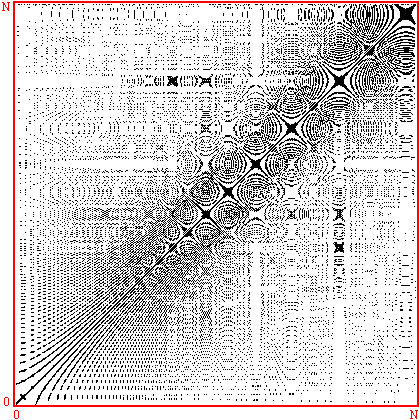The correlation integral is just the "density" of points on a recurrence plot, that is, the number of points divided by the total number of points in the plot.

C(r) = (number of times ||yi - yj|| < r) / N2

Note, the formulation includes the points along the diagonal where i = j. Determining the slope of the correlation integral as a function of log(r) gives the correlation dimension. This is most efficiently computed from a colour recurrence plot where, in general, all the distances have been computed and one simply need to step through a range of radii and count the number of cells with a distance less than the radius.

 In the graph on the right the correlation integral is computed over a range of radii for both a white noise source and the logistic equation operating in the chaotic regime. The embedding dimension is 2 and the delay is 1.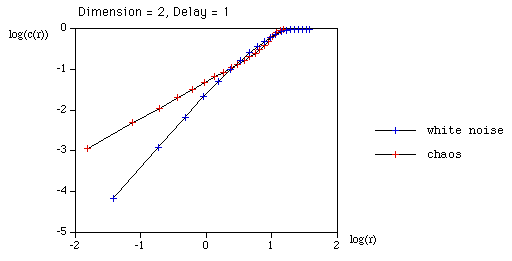The graph on the right uses the same chaotic series as above but varies the embedding dimension from 2 to 6 while keeping the delay fixed at 1.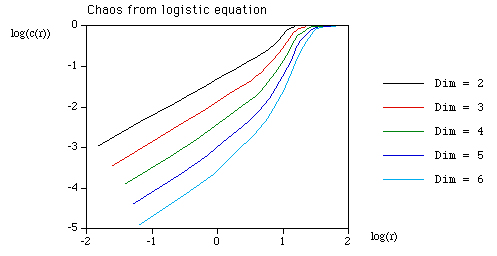In this case the delay is varied from 1 to 3 with a fixed embedding dimension of 2.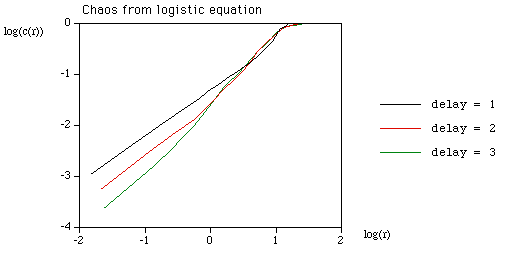The usefulness of this result stems from an observation that the fractal dimension of an attractor of a chaotic system exhibits self similarity. It can be shown that the topological dimension of an embedding is the same as the topological dimension of the underlying attractor as long as the embedding dimension is large enough. Unfortunately there is no straightforward way to determine what "large enough" is except by computing the correlation dimension for a number of different embedding dimensions.
Note, the delay used for the embedding is normally taken to be at the first zero of the auto-correlation function.

Further examples
 Delta function trainSinusoid + noise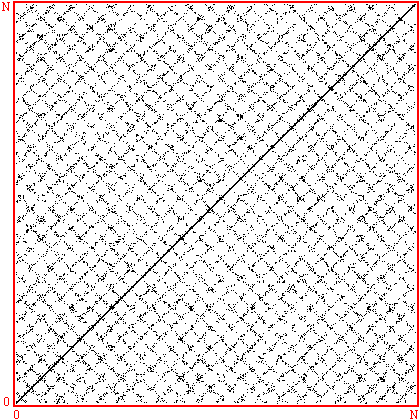Square waveLogistic (double bifurcation) xn = 3.5 * xn-1 ( 1 - xn-1 ) This example also illustrates another small detail, normally one does not wish to make a recurrence map that is as wide and tall as the number of points in the time series. The time series used in these examples is 2000 points wide while the recurrence maps are 400 pixels wide an tall. This is solved by sub-sampling but depending on the dimension and delay there can be a border at the edge of the recurrence plot for which the calculations cannot be performed (see the white fringe on the right and top of this recurrence plot).Decaying sinusoid A change in the thickness of the lines tends to indicate a change in the amplitude of the series.Source code

The following illustrative C source was used to generate the diagrams shown above

References

Understanding Non-linear Dynamics. Daniel Kaplan and Leon Glass. Springer-Verlag

Recurrence Plots of Dynamical Systems. Eckman, J.P., et. al. Europhys. Lett. 4, 973 (1 November 1987).

Characterization of Strange Attractors. Grassberger, P., Procaccia, I. Phys. Rev. Lett. 50, 346 (31 January 1983).

# Self Similarity

Written by Paul Bourke
October 2002, updated June 2007

Fractals usually possess what is called self similarity across scales. That is, as one zooms in or out the geometry/image has a similar (sometimes exact) appearance. The following will illustrate various types of self similarity as well as present some real world examples.

Exact self similarity

The self similarity may be exact, this normally only occurs in mathematically defined fractals where the realities/constraints on structures by the physical world don't apply. The following example is the well known Koch snowflake curve created by starting with a single line segment and on each iteration replacing each line segment by four others shaped as follows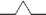. As one successively zooms in the resulting shape is exactly the same no matter how far in the zoom is applied.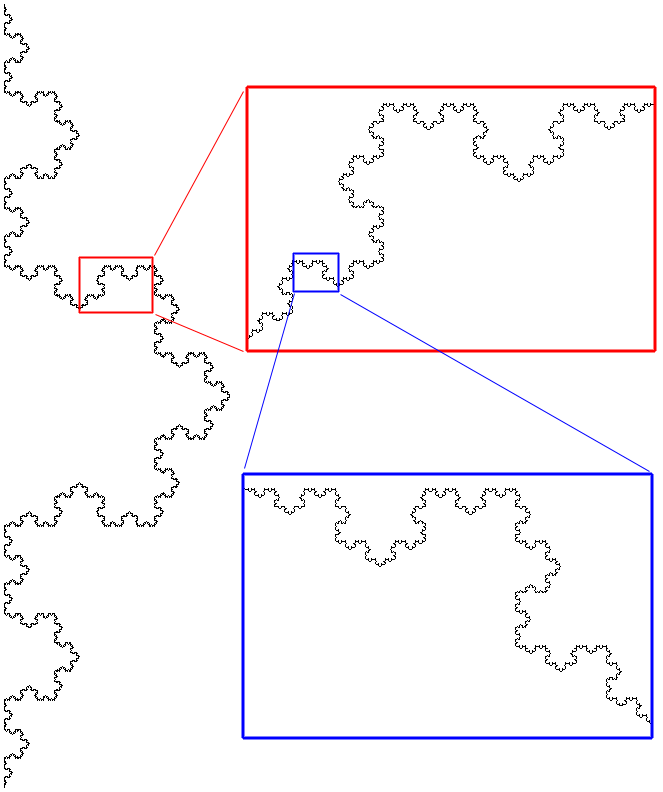Approximate self similarity

A far more common type of self similarity is an approximate one, that is, as one looks at the object at different scales one sees structures that are recognisably similar but not exactly so. An example of this in a mathematically defined system can be readily demonstrated by almost all the patterns seen in the Mandelbrot set. The following show three successive zooms and at each level a structure similar but not exactly the same as the whole Mandelbrot set can be found.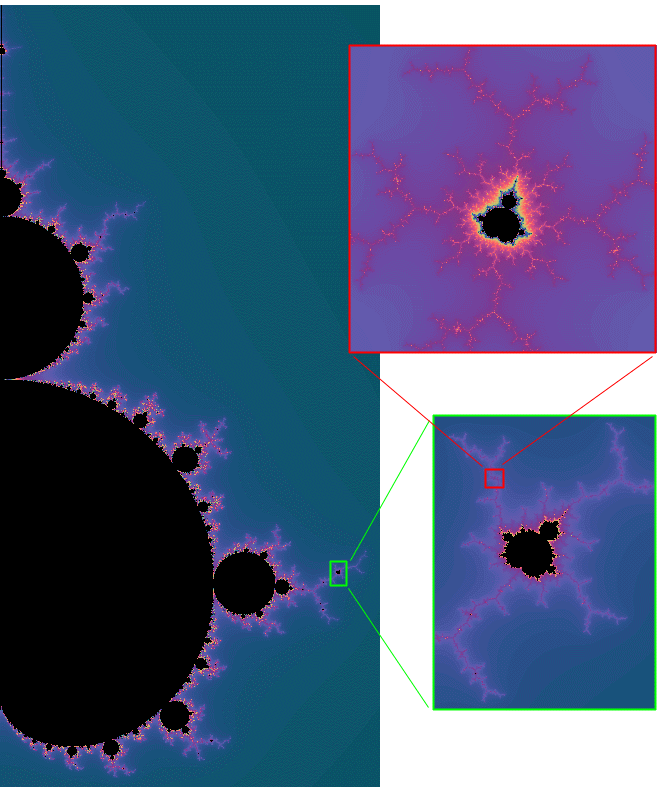Note that in the above example the self similar structure occurs at discrete levels of scale while other systems like the Koch curve the self similarity occurs at all scales.

Statistical self similarity

Sometimes the self similarity is isn't visually obvious but there may be numerical or statistical measures that are preserved across scales. One obvious measure might be the fractal dimension, in the example below of 1/f noise. the fractal dimension is constant as one zooms in.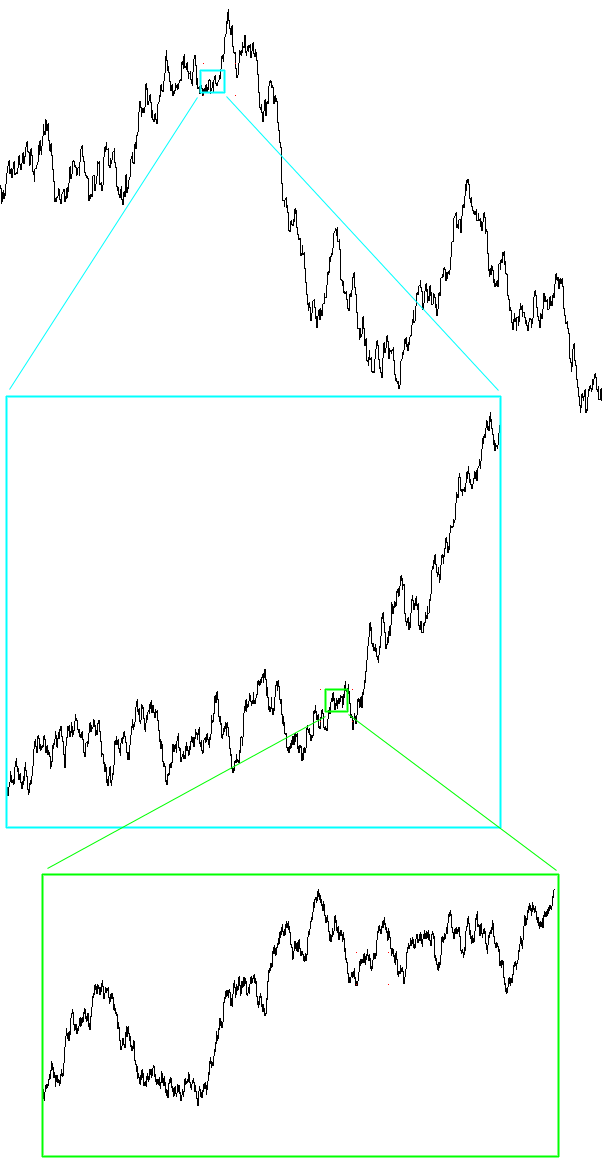Example 1 - Terrain

Consider the following image, is it on the scale of a large piece of rugged terrain photographed from an aeroplane, or the side of a mountain, or a patch of dirt on the scale of a few meters, or a magnification of the surface of a rough rock? Whichever it is, it could also easily be imagined to be any one of the others. So one could start at the large scale view from the air and apply successive zooms down to a microscopic scale, the surface maintains self similarity across those scales.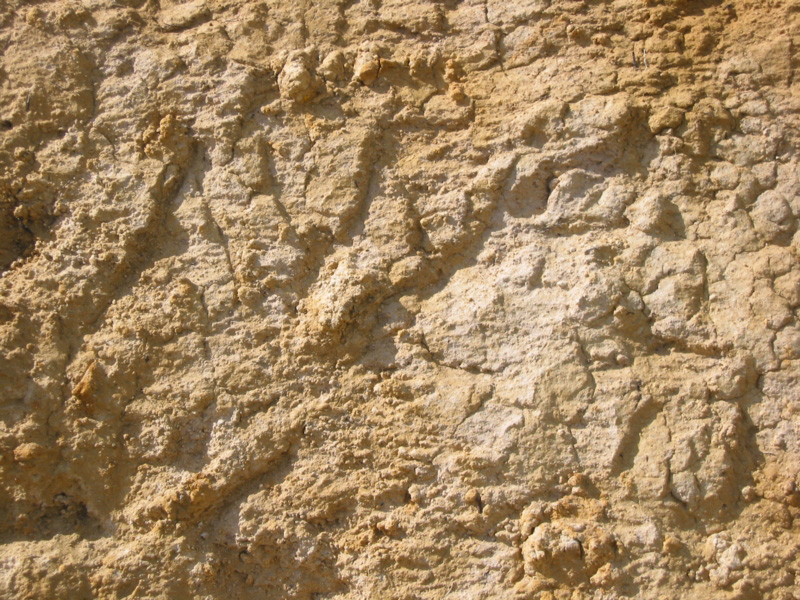As with most fractal structures found in nature, the self similarity only occurs over a range of scales. In the above example, there is no self similarity as we zoom out to see the whole planet, or zoom in to microscopic scales.

Example 2 - Fern leaf

In the case of the self similarity found in the fern, not only is there a limit to the range of scales at which the self similarity occurs but it also occurs at only at a few discrete scales.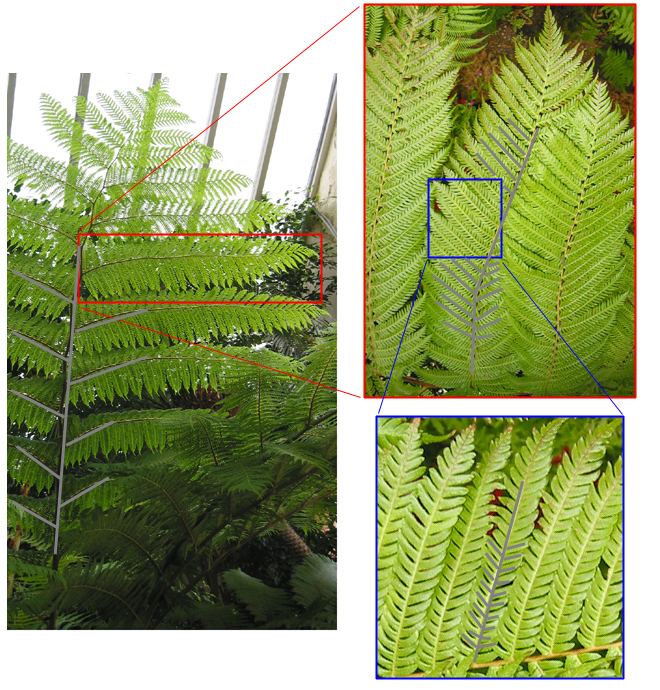Example 3 - Tree branching

Branching structures such as roots, capillaries, river networks often exhibit self similarity across scales. In some cases, a young tree may be self similar in time, namely, as it grows. A Bonsai tree is a good example (if a little artificial) of this. The trees below, besides the fact that you can see them in their context and thus scale, could easily be imagined to be anywhere in size from a small shrub of 10cm, as they are at about 1m tall, and even as small trees of 10 to 20m in height, or large trees of 100m. They would all pass for possible trees across that large range of scales.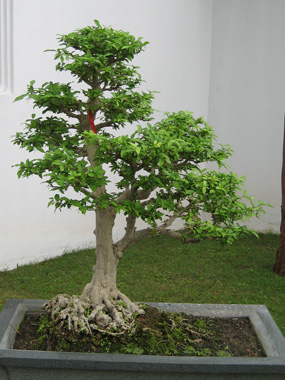Bonsai trees in Chinese gardens, Singapore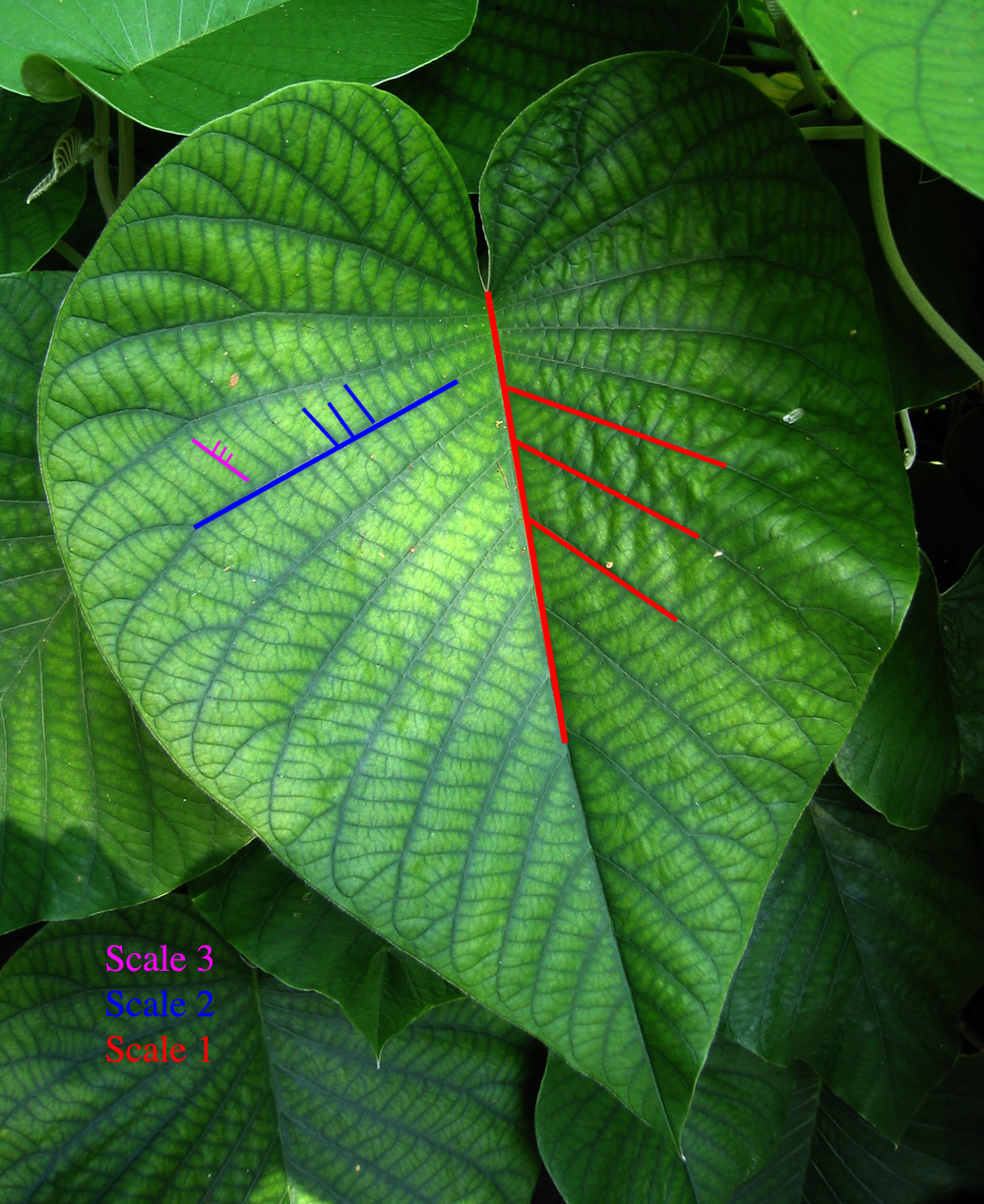Tropical leaf